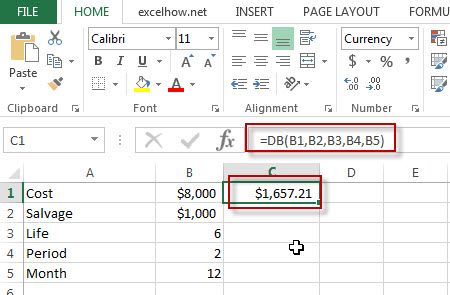## Excel DB Function

This post will guide you how to use Excel DB function with syntax and examples in Microsoft excel.

### Description

The Excel DB function returns the depreciation of an asset for a specified period using the fixed-declining balance method.

The DB function is a build-in function in Microsoft Excel and it is categorized as a Financial Function.

The DB function is available in Excel 2016, Excel 2013, Excel 2010, Excel 2007, Excel 2011 for Mac.

### Syntax

The syntax of the DB function is as below:

`= DB (cost, salvage, life, period, [month])`

Where the DB function arguments are:

• Cost -This is a required argument. The original cost of the asset.
• Salvage – This is a required argument.  The salvage value at the end of the life of the asset.
• Life – This is a required argument.  The number of periods over which the asset is to be depreciated.
• Period – This is a required argument.  The period in which to calculate the depreciation.
• Month – This is an optional argument.  The number of months in the first year of depreciation. The default value is 12.

### Excel DB Function Examples

The below examples will show you how to use Excel DB Function to calculate the depreciation of an asset using the fixed declining balance method.

#1 =DB(B1,B2,B3,B4,B5)### Related Functions

• Excel DDB Function
The Excel DB function returns the depreciation of an asset for a specified period using the double-declining balance method or you can also use some other method to get the depreciation.The syntax of the DDB function is as below:= DDB(cost, salvage, life, period, [factor])…